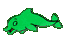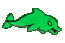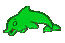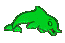Algebra Help - Polynomial Help - Multiply Binomial times Trinomial - Game Tips:

- Distribute each term of the binomial to each term of the trinomial.
- In the example (a+b)(c-d+e) you distribute (multiply) 'a' to get 'ac-ad+ae' and then
you distribute '+b' to get 'bc-bd+be'. So the answer to (a+b)(c-d+e)= ac-ad+ae+bc-bd+be.

- In another example (a-b)(c-d+e) you distribute (multiply) 'a' to get 'ac-ad+ae' and then
you distribute '-b' to get '-bc+bd-be'. So the answer to (a-b)(c-d+e)= ac-ad+ae-bc+bd-be.

- Your Game Score is reduced by the number of dolphin hits.

- To slow the game speed repeat tap/click on the word Slider.
- To increase the game speed repeat tap/click on the word Math.
- Speed can also be adjusted with a keyboard's - and + keys.

- Refresh/Reload the web page to restart the game.